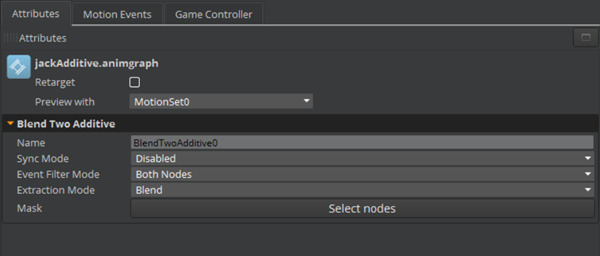The Blend Two Additive node blends a pose 2 input additively on top of a pose 1 input.Note:
This node functions similarly to the Blend Two (Legacy) node with Additive enabled. The key difference is that the Blend Two (Legacy) node subtracts the bind pose when applying an additive blend. Furthermore, Blend Two Additive expects pose 2 to be an additive pose. This means that if you wanted Blend Two Additive to function like the Blend Two (Legacy) node, you must first subtract the bind pose from pose 2.

To use the Blend Two Additive node

1. In O3DE Editor, choose Tools, Animation Editor.

2. Create a blend tree .

3. Double-click the blend tree node that you created.

4. Select the Anim Graph Palette tab and then select the Blending tab.

5. Drag the Blend Two Additive node into the animation graph.Connect nodes to the following inputs and output:

• Pose 1 - The base pose.

• Pose 2 - The pose to be added to Pose 1.

• Weight - Weight of additive.

You can use a Float Constant node, for example, to specify a float value between `0.0` and `1.0`. A value of `0.0` means Pose 2 does not affect Pose 1 at all. A value of `1.0` means that Pose 2 is fully added on top of Pose 1. Other nodes that can specify Weight include the Parameter node, the Smoothing node, and so on.

• Output Pose - The result of the blended poses, which you can visualize as `Pose 1 + (Pose 2 * Weight)`.

## Blend Two Additive Node Attributes

For attribute settings that are shared among the blend node types, see Blend Node Attributes .

The output from motion extraction with the Blend Two Additive node is calculated as follows.

• `S` = Source transform delta
• `T` = Target transform delta

• Blend = `S` + `T` * weight
• Source = `S`
• Target = `T`
• Blend = `S`
• Source = `S`
• Target = `S`